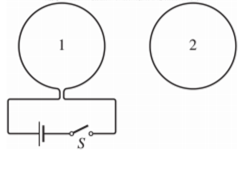# Problem: Two loops of wire are next to each other in the same plane.(a) If the switch S is close, what is the direction of current in loop 2?(b) What is the direction of the magnetic force on loop 2?(c) Loop 1 is moved horizontally to the left. What is the direction of current in loop 2?

###### FREE Expert Solution

(a)

If the switch is closed, the current will start flowing in loop 1 in a clockwise direction.

The current in the loop 1 creates a magnetic field, which is directed out of the page in loop 2.

As the current is increasing, the magnetic field strength also increases.

The current is then inducted in loop 2.

91% (5 ratings)###### Problem Details

Two loops of wire are next to each other in the same plane.
(a) If the switch S is close, what is the direction of current in loop 2?
(b) What is the direction of the magnetic force on loop 2?
(c) Loop 1 is moved horizontally to the left. What is the direction of current in loop 2?